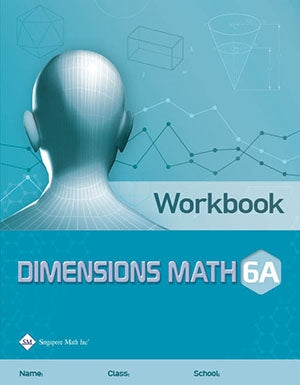# Singapore Math Dimensions Math Workbook 6A

Workbooks are the essential supplements to textbooks as they give students the opportunity to practice applying new concepts. Workbook exercises polish analytical skills and help students develop a stronger foundation in math through repetition and variation.

Features & Components:

• Basic Practice: Simple questions that drill comprehension of concepts.
• Further Practice: More difficult questions that involve direct application.
• Challenging Practice: Questions that require synthesis.

The answer key at the back of the book provides answers only. Workbook Solutions (sold separately) contain fully worked solutions.

Note: Two workbooks (A and B) correspond to the two halves of the school year. Soft cover.

Dimensions Math® Workbook 6A

Chapter 1: Whole Numbers

1.1 Order of Operations

A. Expressions and Equations
B. Exponents
C. Order of Operations without Parentheses
D. Order of Operations with Parentheses

1.2 Factors and Multiples

A. Factors
B. Multiples

1.3 Multiplication

A. Properties of Multiplication
B. Mental Multiplication

1.4 Division

A. Properties of Division
B. Mental Division

In A Nutshell
Problem Solving Corner

Chapter 2: Fractions

2.1 Multiplication of Fractions

A. Multiplication of a Proper Fraction by a Whole Number
B. Multiplication of a Proper Fraction by a Fraction
C. Multiplication of an Improper Fraction or a Mixed Number by a Whole Number
D. Multiplication of an Improper Fraction or a Mixed Number by a Fraction

2.2 Division of Fractions

A. Division of a Whole Number by a Fraction
B. Division of a Fraction by a Whole Number
C. Division of a Fraction by a Fraction

In A Nutshell
Problem Solving Corner

Chapter 3: Decimals

3.1 Addition and Subtraction of Decimals

A. Structure of Decimals

3.2 Multiplication of Decimals

A. Decimal Number System
B. Multiplier and Product
C. Multiplying Decimals

3.3 Division of Decimals

A. Divisor and Quotient
B. Dividing Decimals

3.4 Metric Measurements and Decimals

In A Nutshell
Problem Solving Corner

Chapter 4: Negative Numbers

4.1 Positive and Negative Numbers
4.2 Comparing Positive and Negative Numbers

A. The Number Line
B. Absolute Value

In A Nutshell

Chapter 5: Ratios

5.1 Ratios and Equivalent Ratios

A. Finding Ratio
B. Equivalent Ratios

5.2 Ratios and Fractions

In A Nutshell
Problem Solving Corner

Chapter 6: Rate

6.1 Average and Rate
6.2 Unit Rate
6.3 Speed

In A Nutshell
Problem Solving Corner

Chapter 7: Percent

7.1 Meaning of Percent
7.2 Percentage of a Quantity

In A Nutshell